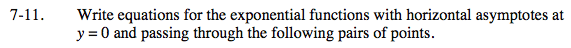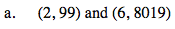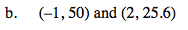### Home > INT3 > Chapter 7 > Lesson 7.1.1 > Problem7-11

7-11.
1. Write equations for the exponential functions with horizontal asymptotes at y = 0 and passing through the following pairs of points. Homework Help ✎

1. (2, 99) and (6, 8019)

2. (–1, 50) and (2, 25.6)Use the points to write and solve a system of equations of the form y = abx.

99 = ab2
8019 = ab6$y=40(0.8)^x$